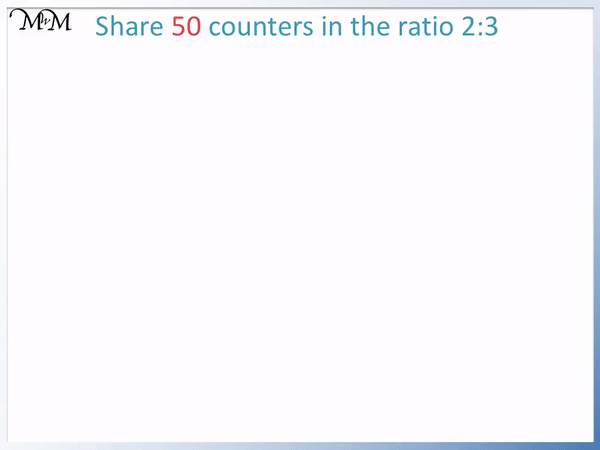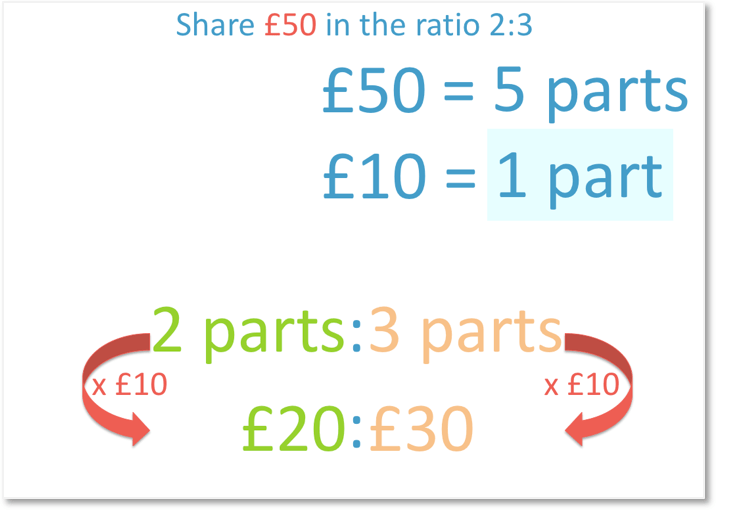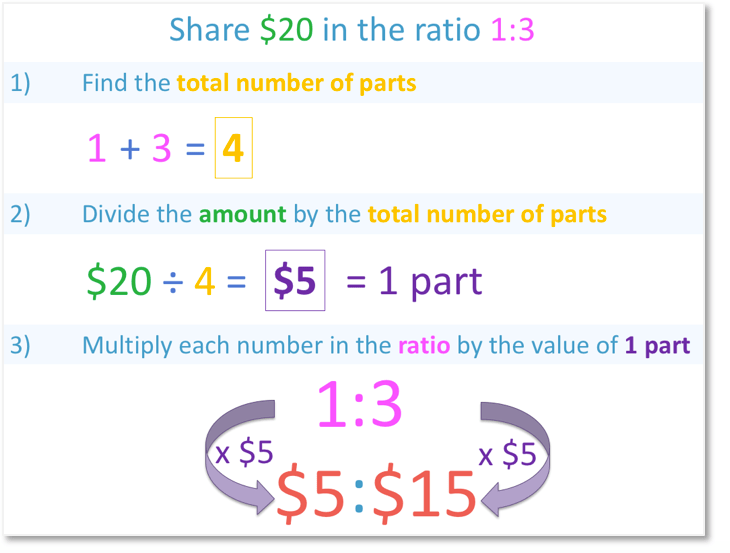# Solving Ratio Problems

Solving Ratio Problems• We add the parts of the ratio to find the total number of parts.
• There are 2 + 3 = 5 parts in the ratio in total.
• To find the value of one part we divide the total amount by the total number of parts.
• 50 ÷ 5 = 10.
• We multiply the ratio by the value of each part.
• 2:3 multiplied by 10 gives us 20:30.
• The 50 counters are shared into 20 counters to 30 counters.
Find the value of one part of the ratio by dividing the amount by the total number of parts.

Multiply the ratio by the value of one part.• 2 + 3 = 5 and so there are 5 parts in the ratio in total.
• We divide by this total number of parts to find the value of each part.
• 50 ÷ 5 = 10.
• We multiply the original ratio by the value of each part.
• We have 20:30.Supporting Lessons# How to Solve Ratio Problems

Here is an example of a ratio problem. In this lesson we will look at how we solve ratio problems using a few examples.We are asked to share £50 in the ratio 2:3.

This means that for every two parts a person receives, a second person is given three parts.We begin by finding the total number of parts.

There are two parts highlighted in green and three parts highlighted in orange.We have five parts in our ratio in total.In total, all five parts are worth £50.

To be able to find out how much money each person is given, we need to know how much one part is worth.Each part in the ratio must have the same value.

This means that £50 must be shared equally over the five parts.

To share equally, we divide £50 by 5.

50 ÷ 5 = 10

So, each part is worth £10.Now that we know that one part is worth £10, we can find out what two parts and three parts are worth.If one part is £10, two parts must equal £20.

£10 x 2 = £20

If one part is £10, three parts must equal £30.

£10 x 3 = £30

The money in this ratio problem has been shared £20:£30.

One person receives £20 and the other receives £30. We can check our answer by adding the two amounts to see if they make our original total.

£20 + £30 = £50Now try our lesson on How to Calculate a Ratio of a Number where we break down the process of sharing in a ratio into three steps.